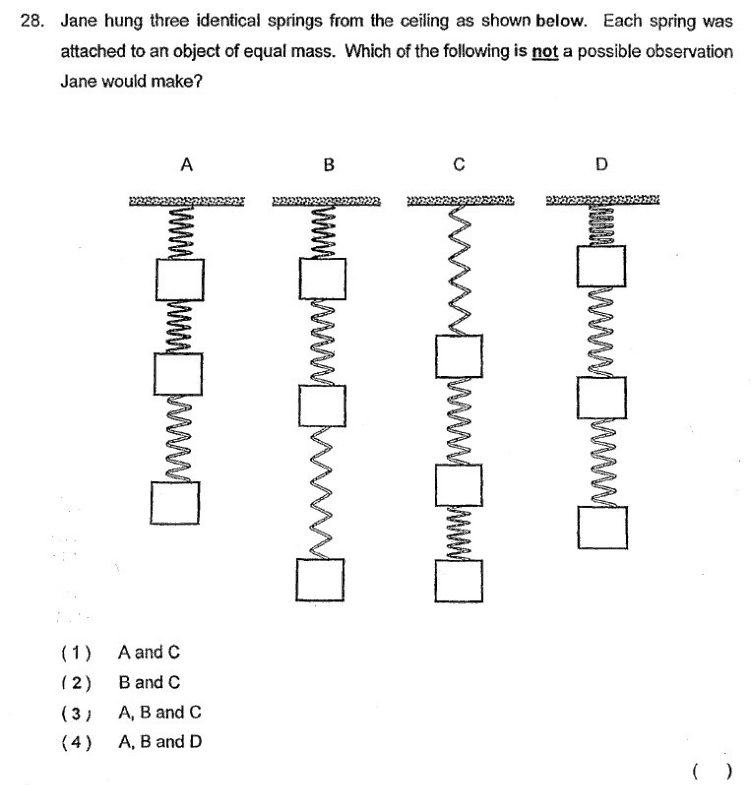# QuestionCan I know the answer for this question and why? Thanks in advance!

1 Answer

# Answer

Answer is (4).

Concept 1: Since the springs are identical, this means the elasticity of the springs is also identical.

Concept 2: The springs above are subject to more gravitational force as there are more weights attached below them. The greater the gravitational force, the greater the stretch of the spring.

Based on the two concepts outlined above, this means that it is impossible for the top springs to be stretched lesser than the springs below it, since they are subject to the most weight. Therefore, A, B, and D are impossible.

1 Reply 0 Likes ✔Accepted Answer

Thanks for the detailed answer!

0 Replies 0 Likes